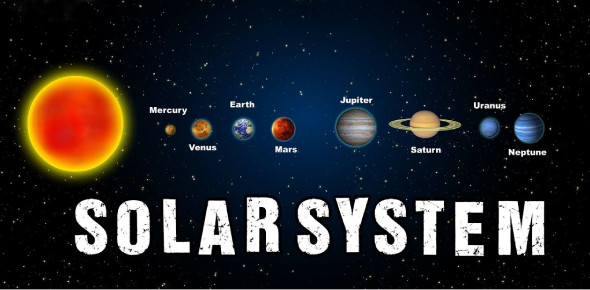# The Solar System Trivia Quiz: Ultimate Facts

15 Questions | Total Attempts: 53Settings.

• 1.
The earth and the moon are alike in that both
• A.

Have liquid water

• B.

Have satellites

• C.

Have an atmosphere

• D.

• 2.
The three main types of landforms that make of the moon's surface are
• A.

Craters, equators, and gyrators

• B.

Volcanoes, highlands, and eclipses

• C.

Marias, santas, and domes

• D.

Highlands, craters, and marias

• 3.
The moon            in a close path around the earth.
• A.

Revolves

• B.

Comets

• C.

Rotates

• D.

Orbit

• 4.
The moon's path around the Earth is called its                .
• A.

Comets

• B.

Satellite

• C.

Orbit

• D.

Axis

• 5.
The Earth is the                largest planet.
• A.

First

• B.

Fourth

• C.

Sixth

• D.

Fifth

• 6.
All nine planets in our solar system orbit around the sun.
• A.

True

• B.

False

• 7.
The Earth takes about            year to orbit around the sun.
• A.

One

• B.

Two

• C.

Three

• D.

Ten

• 8.
Some planets are made up of gasses and liquid.
• A.

True

• B.

False

• 9.
Saturn is not surrounded by a big flat ring.
• A.

True

• B.

False

• 10.
What is a planet?
• 11.
Can you name the planets in order starting from the sun?
• 12.
The biggest planet is             ?
• A.

Uranus

• B.

Pluto

• C.

Jupiter

• D.

Saturn

• 13.
What is the length of time it takes for the Earth to rotate around on its axis 360 degrees?
• A.

50 minutes

• B.

1 year

• C.

30 days

• D.

24 hours

• 14.
A                              is when the moon goes between the Earth and the Sun, and its shadow hits the Earth.
• A.

Lunar eclipse

• B.

Rotating Earth

• C.

Solar Eclipse

• D.

Never happens

• 15.
A                                is when the moon is in the Earth's shadow so the moon is eclipsed by the Earth.
• A.

Rotating axis

• B.

Orbiting Earth

• C.

Solar Eclipse

• D.

Lunar Eclipse

Related TopicsBack to top